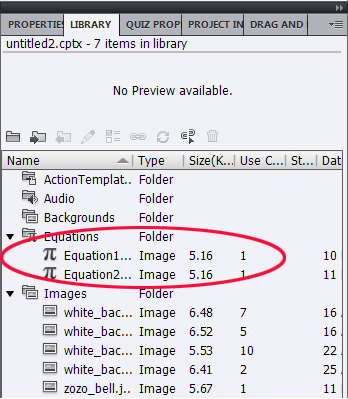Adobe Captivate is integrated with MathMagic™ to let you create and edit Mathematical equations in your courses. MathMagic is a multi-purpose equation editor, formula editor, and a scientific symbol editor for various math formats.

The MathMagic equations are stored as a PNG resource in Adobe Captivate. In the Adobe Captivate library, the equations are categorized under Equations.MathMagic equations in the Adobe Captivate library

## Create equations

1.  Click Insert > Equation. The default equation is inserted into the slide and MathMagic opens automatically.

2. Edit the equation in MathMagic as required. For more information, see MathMagic help.

3. Click File > Save to change the changes to the equation in MathMagic.

The changes are automatically reflected in your Adobe Captivate slide.

## Edit equations

1. Double-click the equation that you want to edit. The equation is opened in MathMagic.

2. Edit the equation as required. For more information, see MathMagic help.

3. Click File > Save to change the changes to the equation in MathMagic.

The changes are automatically reflected in your Adobe Captivate slide.

## Delete equations

Click the equation and press the Delete key.

## Properties of equations

An equation is a noninteractive object in Adobe Captivate, and you can set the following properties for equations:

• Shadow and reflection
• Timing
• Transition
• Audio
• Transform (position, size, and rotate)

For more information, see Adding and managing objects.Twitter™ and Facebook posts are not covered under the terms of Creative Commons.